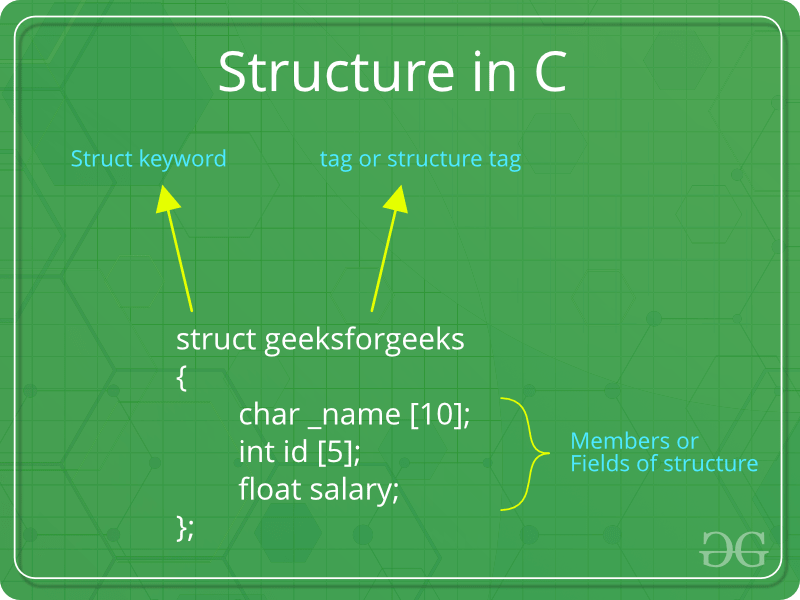# Structures in C

• Difficulty Level : Easy
• Last Updated : 13 Feb, 2022

What is a structure?
A structure is a key word that create user defined data type in C/C++. A structure creates a data type that can be used to group items of possibly different types into a single type.How to create a structure?
‘struct’ keyword is used to create a structure. Following is an example.

## C

 `struct` `address``{``   ``char` `name;``   ``char` `street;``   ``char` `city;``   ``char` `state;``   ``int` `pin;``};`

How to declare structure variables?
A structure variable can either be declared with structure declaration or as a separate declaration like basic types.

## C

 `// A variable declaration with structure declaration.``struct` `Point``{``   ``int` `x, y;``} p1;  ``// The variable p1 is declared with 'Point'`  `// A variable declaration like basic data types``struct` `Point``{``   ``int` `x, y;``};` `int` `main()``{``   ``struct` `Point p1;  ``// The variable p1 is declared like a normal variable``}`

Note: In C++, the struct keyword is optional before in declaration of a variable. In C, it is mandatory.

How to initialize structure members?
Structure members cannot be initialized with declaration. For example the following C program fails in compilation.

## C

 `struct` `Point``{``   ``int` `x = 0;  ``// COMPILER ERROR:  cannot initialize members here``   ``int` `y = 0;  ``// COMPILER ERROR:  cannot initialize members here``};`

The reason for above error is simple, when a datatype is declared, no memory is allocated for it. Memory is allocated only when variables are created.
Structure members can be initialized using curly braces ‘{}’. For example, following is a valid initialization.

## C

 `struct` `Point``{``   ``int` `x, y;``};` `int` `main()``{``   ``// A valid initialization. member x gets value 0 and y``   ``// gets value 1.  The order of declaration is followed.``   ``struct` `Point p1 = {0, 1};``}`

How to access structure elements?
Structure members are accessed using dot (.) operator.

## C

 `#include` `struct` `Point``{``   ``int` `x, y;``};` `int` `main()``{``   ``struct` `Point p1 = {0, 1};` `   ``// Accessing members of point p1``   ``p1.x = 20;``   ``printf` `("x = %d, y = %d", p1.x, p1.y);` `   ``return` `0;``}`
Output:
`x = 20, y = 1`

What is designated Initialization?
Designated Initialization allows structure members to be initialized in any order. This feature has been added in C99 standard

## C

 `#include` `struct` `Point``{``   ``int` `x, y, z;``};` `int` `main()``{``   ``// Examples of initialization using designated initialization``   ``struct` `Point p1 = {.y = 0, .z = 1, .x = 2};``   ``struct` `Point p2 = {.x = 20};` `   ``printf` `("x = %d, y = %d, z = %d\n", p1.x, p1.y, p1.z);``   ``printf` `("x = %d", p2.x);``   ``return` `0;``}`
Output:
```x = 2, y = 0, z = 1
x = 20```

This feature is not available in C++ and works only in C.

What is an array of structures?
Like other primitive data types, we can create an array of structures.

## C

 `#include` `struct` `Point``{``   ``int` `x, y;``};` `int` `main()``{``   ``// Create an array of structures``   ``struct` `Point arr;` `   ``// Access array members``   ``arr.x = 10;``   ``arr.y = 20;` `   ``printf``("%d %d", arr.x, arr.y);``   ``return` `0;``}`
Output:
`10 20`

What is a structure pointer?
Like primitive types, we can have pointer to a structure. If we have a pointer to structure, members are accessed using arrow ( -> ) operator.

## C

 `#include` `struct` `Point``{``   ``int` `x, y;``};` `int` `main()``{``   ``struct` `Point p1 = {1, 2};` `   ``// p2 is a pointer to structure p1``   ``struct` `Point *p2 = &p1;` `   ``// Accessing structure members using structure pointer``   ``printf``("%d %d", p2->x, p2->y);``   ``return` `0;``}`
Output:
`1 2`

What is structure member alignment?
Limitations of C Structures
In C language, Structures provide a method for packing together data of different types. A Structure is a helpful tool to handle a group of logically related data items. However, C structures have some limitations.

• The C structure does not allow the struct data type to be treated like built-in data types:

• We cannot use operators like +,- etc. on Structure variables. For example, consider the following code:

## C

 `struct` `number``{``    ``float` `x;``};``int` `main()``{``    ``struct` `number n1,n2,n3;``    ``n1.x=4;``    ``n2.x=3;``    ``n3=n1+n2;``    ``return` `0;``}` `/*Output:` `prog.c: In function 'main':``prog.c:10:7: error:``invalid operands to binary + (have 'struct number' and 'struct number')``  ``n3=n1+n2;` `*/`

• No Data Hiding: C Structures do not permit data hiding. Structure members can be accessed by any function, anywhere in the scope of the Structure.

• Functions inside Structure: C structures do not permit functions inside Structure

• Static Members: C Structures cannot have static members inside their body

• Access Modifiers: C Programming language do not support access modifiers. So they cannot be used in C Structures.

• Construction creation in Structure: Structures in C cannot have constructor inside Structures.

Related Article : C Structures vs C++ Structures

We will soon be discussing union and other struct related topics in C. Please write comments if you find anything incorrect, or you want to share more information about the topic discussed above.

My Personal Notes arrow_drop_up